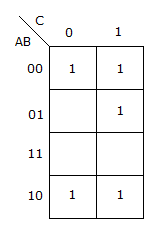# Electronics and Communication Engineering - Digital Electronics

16.

2's complement representation of a 16 bit number (one sign bit and 15 magnitude bits) is FFFF. Its magnitude in decimal representation is

 A. 0 B. 1 C. 32, 767 D. 65, 535

Explanation:

No answer description available for this question. Let us discuss.

17.

The method used to transfer data from I/O units to memory by suspending the memory-CPU data transfer for one memory cycle is called

 A. I/O spooling B. Cycle stealing C. Line conditioning D. Demand paging

Explanation:

No answer description available for this question. Let us discuss.

18.

Out of SAM and RAM

 A. access time in both does not depend on address location B. in SAM access time depends on address location but not in RAM C. in RAM access time depends on address location but not in SAM D. in both the access time depends on storage location

Explanation:

No answer description available for this question. Let us discuss.

19.

Bipolar IC memories are fabricated using

 A. high density versions of MOSFET or low density versions of bipolar transistor flip-flop. B. high density version of MOSFET or bipolar transistor flip-flop C. low density versions of MOSFETs D. high density versions of the bipolar transistor flip-flop.

Explanation:

No answer description available for this question. Let us discuss.

20.

For K map of the given figure the simplified Boolean expression isA. Y = A B C + A B C + A B C B. Y = A C + B C. Y = A B C + ABC D. Y = A B C + AB C + ABC